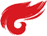算法第二章上机实践报告

1.实践题目名称

2.问题描述

7-1 最大子列和问题 (20分)

• 数据1：与样例等价，测试基本正确性；
• 数据2：102个随机整数；
• 数据3：103个随机整数；
• 数据4：104个随机整数；
• 数据5：105个随机整数；

### 输入样例:

6
-2 11 -4 13 -5 -2

### 输出样例:

20

3.算法描述

本题需要利用分治法分解主问题，对于本问题，在暴力穷举的算法的思想上进行优化，把所求区间从中间一分为二，将问题划分为求左区间、右区间、横跨左右区间的最大子列和的问题。左右区间的求解利用基础的递归方式完成，中间的最大子列和另外做特殊处理，最后将所求的三者进行对比得到答案，这种方式能优化时间复杂度到nlog(n)，大大提高效率。

#include<bits/stdc++.h>
using namespace std;
int three_max(int a,int b,int c){
if(a>b&&a>c) return a;
if(b>a&&b>c) return b;
if(c>a&&c>b) return c;
}
int max_subsequence(int sum[],int left,int right){
int max_left=0,max_right=0;
int max_border_left=0,max_border_right=0;
int sum_left=0,sum_right=0;
if(left==right) return 0>sum[left]?0:sum[left];
int mid=(left+right)/2;
max_left=max_subsequence(sum,left,mid);
max_right=max_subsequence(sum,mid+1,right);
for(int i=mid;i>=left;--i){
sum_left+=sum[i];
if(sum_left>max_border_left) max_border_left=sum_left;
}
for(int i=mid+1;i<=right;++i){
sum_right+=sum[i];
if(sum_right>max_border_right) max_border_right=sum_right;
}
return three_max(max_border_left+max_border_right,max_left,max_right);
}
int main(){
int k;
cin>>k;
int sum[k];
for(int i=0;i<k;++i){
cin>>sum[i];
}
cout<<max_subsequence(sum,0,k-1);
return 0;
}

4.算法时间复杂度分析

设算法的复杂度为T(N)，

第一步分解为两个子问题为O(1)

第二步分别求解两个子问题为O(N/2)*2

第三步合并子问题为O(N)

等式为：T(N)=O(1)+2T(N/2)+O(N)

得：T(N)=O（nlogn）

5.心得体会（对本次实践收获及疑惑进行总结）

本次的题目就我看来算是一道分治法的经典例题，虽然一开始我并不能理解为什么一定要分而治之，因为在我看来都是要遍历一遍所有数据，但是后来我逐渐想通了这种方法能节省的时间，也深刻的认识到好算法的重要性。

posted on 2020-10-08 18:47  我也想承認我是傲嬌  阅读(118)  评论(0编辑  收藏  举报